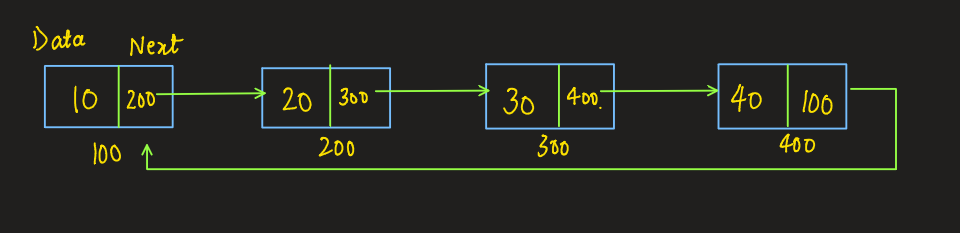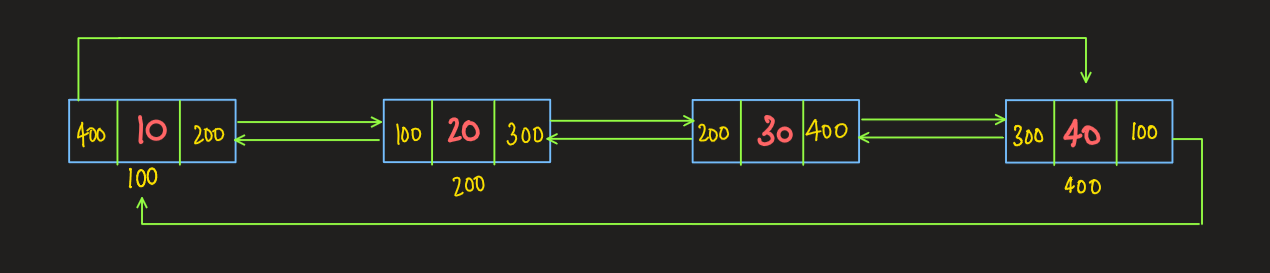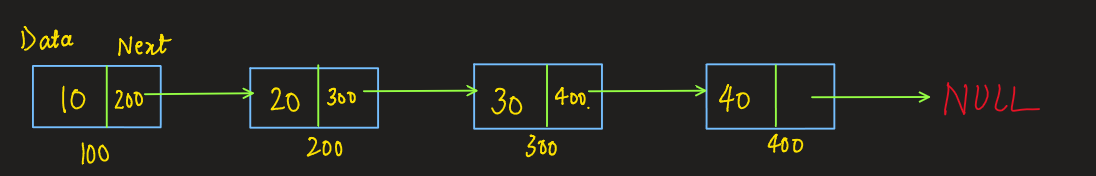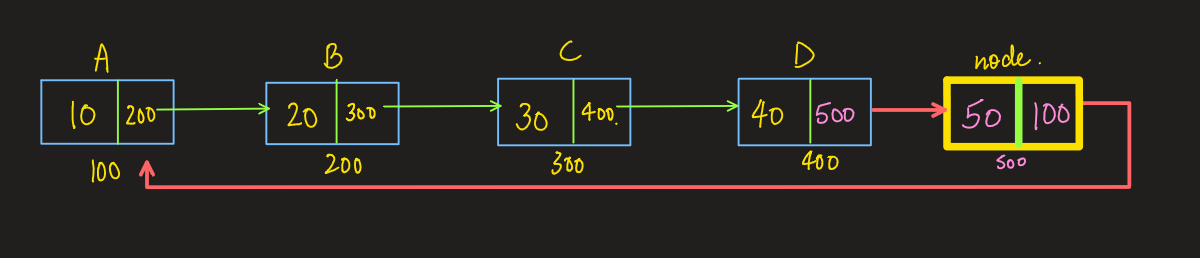# Circular Linked List in C++

Pre-requisite: Singly Linked Lists

## What is a Circular Linked List?

As the name suggest in a circular linked list, the last node of the linked list points to first node forming a circle. That is why it is called a circular linked list. A circular linked list is same as normal linked list except for the facts that no node in a circular linked list points to NULL as the last node points back to the first node.

It can be divided into two types:

• Singly Circular Linked List
• Doubly Circular Linked List

The following image depicts the structure of a singly circular linked listThe following image depicts the structure of a doubly circular linked list### Operations On Circular Linked List:

There are various operations that can be performed on a circular linked list. Some of the operations are listed below.

1. Insertion
1. At beginning
2. At the end
2. Deletion
1. From beginning
2. From the end
3. Traversal
4. Searching

## Implementing a Circular Linked List

To implement a circular linked list, we use a singly linked list and add a note at the end of the linked list which would point to the first node of the linked list.

Suppose we have a singly linked list as followsAt the end of this linked list, we add a new node with the name “node”, later we make that node point to the first node. The following diagram depicts the procedure.### Insertion in a singly circular linked list:

Insertion at the beginning of a linked list:

We can insert at the beginning of CLL in O(1) and O(n) time complexity.

To achieve that, we iterate to the end of the CLL, and once we reach the last node we perform the following steps:

1. We make the next pointer of our newnode to point to the next of our last node
2. Change the next pointer of our last node to point it to the new node.
3. Return the new node [new head of your CLL]

To do this in O(1) time complexity, simply make the newnode the second node of your CLL and swap the data of the head node and the new node.

Code:

## C++ Code

``````node * insertion_beg(node * head, int x) {
node * newnode = new node(x);
if (head == NULL) { // CLL is empty
newnode -> next = newnode;
return newnode;
} else {
//   O(n) approach
//       current=current->next;
//   }
//   newnode->next=current->next;
//   current->next=newnode;
//   return newnode;

// O(1) approach
}
}
``````

Insertion at end

Approach: This is very similar to insertion at the beginning, the difference here is, we are returning the original head as our head of CLL.

To do this, traverse to the end of CLL and:

1. Change the next pointer of your last node to point to the new node.
2. Change the next pointer of your new node to point to the head of the CLL.

Code:

## C++ Code

``````node * insertion_end(node * head, int x) {
node * newnode = new node(x);
if (head == NULL) {
newnode -> next = newnode;
return newnode;
}
else {
node * current = head;
while (current -> next != head) {
current = current -> next;
}
current -> next = newnode;
newnode -> next = head;
}
}
``````

## Deletion in Circular Linked Lists

Deletion from beginning

Approach: This can be done in both O(1) and O(n) time complexity.

Simply iterate to the end of your CLL and

1. Change the next pointer of your last node to point to the next of your head node
2. Delete the head.
3. Return the next of your last node as the new head of CLL

To do this in O(1) time complexity,

1. Copy the data of your second node into the head node
2. Store the second node in a temporary node
3. Change the next pointer of your head node to point to the third node of your CLL
4. Delete the temporary node.
5. Return the new head.

Code:

## C++ Code

``````node * deletion_beg(node * head) {
if (head == NULL) return NULL; //checking if list is empty

if (head -> next == head) {
return NULL;
}
// O(n) approach
//   while(current->next!=head){ // iterating to the last node
//     current=current->next;
//   }
//   current->next=head->next; // changing the next of last node to point to second node

//   return current->next;

//O(1) approach
head -> data = head -> next -> data;
node * temp = head -> next;
head -> next = head -> next -> next;
delete temp;
}
``````

Deletion from end

Approach: To perform this, we need to keep the track of our last node and the second last node of our CLL. To achieve this we use 2 nodes, one as current and the other as prevNode.

1. Iterate to the end of CLL and store the second last node and last node in prevNode and current respectively.
2. Change the prevNode’s next pointer to point to the current’s next i.e. head.
3. Delete current.

Code:

## C++ Code

``````node * deletion_end(node * head) {
if (head == NULL) return NULL;
if (head -> next == head) {
return NULL;
}

node * prevNode; // to keep the track of previous node
node * current = head;
while (current -> next != head) {
prevNode = current;
current = current -> next;
}
prevNode -> next = current -> next; // changing the second last node’s next
to point to last node’s next node [head]
delete(current);
}
``````

### Searching in Circular Linked List

Approach: We iterate through the CLL and check if the current node’s data matches the target data. If it matches, we mark the flag as true and print “Found” else we print “Not Found”.

Code:

## C++ Code

``````bool searching(node * head, int x) {
node * current = head;
bool flag = false;
do{
if(current->data==x){
flag=true; // if found, marking flag=true and breaking out of the loop
break;
}
current=current->next;
return flag;
}
``````

### Traversal in Circular Linked List:

Approach: The idea here is to mark the head as the current node and move it until it reaches back to the head since we don’t have NULL in CLL. We print the elements to check if the iteration is correct.

Code:

## C++ Code

``````void traversal(node * head) {
node * current = head;
do {
cout << current -> data << " ";
current = current -> next;
} while (current != head);
}
``````

Uses of Circular Linked List

A circular linked list has many practical uses. Some of the uses are listed below

1. In our web browsers, multiple tabs are running simultaneously and browsers provide us with the feature of switching between the tabs which is achieved by circular linked list.
2. Circular linked list can be used to implement data structures like circular queues, etc.
3. Multiplayer games use circular linked lists to equally divide and assign turns to the players.
4. A circular linked list can be used to implement a round-robin scheduling algorithm.

Special thanks to Yash Mishra for contributing to this article on takeUforward. If you also wish to share your knowledge with the takeUforward fam, please check out this article